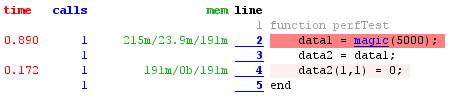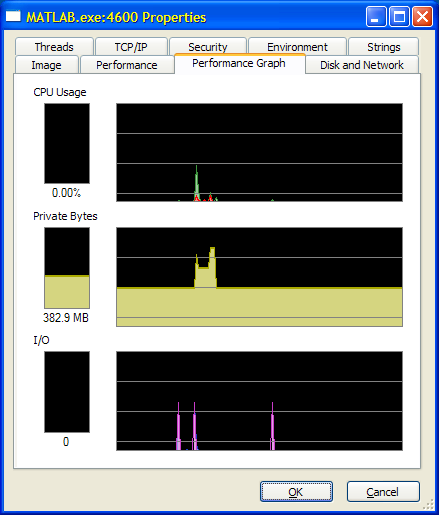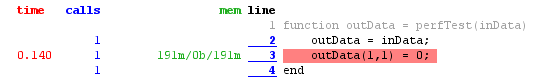# Internal Matlab memory optimizations

Yesterday I attended a seminar on developing trading strategies using Matlab. This is of interest to me because of my IB-Matlab product, and since many of my clients are traders in the financial sector. In the seminar, the issue of memory and performance naturally arose. It seemed to me that there was some confusion with regards to Matlab’s built-in memory optimizations. Since I discussed related topics in the past two weeks (preallocation performance, array resizing performance), these internal optimizations seemed a natural topic for today’s article.

The specific mechanisms I’ll describe today are Copy on Write (aka COW or Lazy Copying) and in-place data manipulations. Both mechanisms were already documented (for example, on Loren’s blog or on this blog). But apparently, they are still not well known. Understanting them could help Matlab users modify their code to improve performance and reduce memory consumption. So although this article is not entirely “undocumented”, I’ll give myself some slack today.

### Copy on Write (COW, Lazy Copy)

Matlab implements an automatic copy-on-write (sometimes called copy-on-update or lazy copying) mechanism, which transparently allocates a temporary copy of the data only when it sees that the input data is modified. This improves run-time performance by delaying actual memory block allocation until absolutely necessary. COW has two variants: during regular variable copy operations, and when passing data as input parameters into a function:

#### 1. Regular variable copies

When a variable is copied, as long as the data is not modified, both variables actually use the same shared memory block. The data is only copied onto a newly-allocated memory block when one of the variables is modified. The modified variable is assigned the newly-allocated block of memory, which is initialized with the values in the shared memory block before being updated:

```data1 = magic(5000); % 5Kx5K elements = 191 MB data2 = data1; % data1 & data2 share memory; no allocation done data2(1,1) = 0; % data2 allocated, copied and only then modified```

If we profile our code using any of Matlab’s memory-profiling options, we will see that the copy operation `data2=data1` takes negligible time to run and allocates no memory. On the other hand, the simple update operation `data2(1,1)=0`, which we could otherwise have assumed to take minimal time and memory, actually takes a relatively long time and allocates 191 MB of memory.Copy-on-write effect monitored using the Profiler's -memory optionCopy-on-write effect monitored using Windows Process Explorer

We first see a memory spike (used during the computation of the magic square data), closely followed by a leveling off at 190.7MB above the baseline (this is due to allocation of data1). Copying `data2=data1` has no discernible effect on either CPU or memory. Only when we set `data2(1,1)=0` does the CPU return, in order to allocate the extra 190MB for data2. When we exit the test function, data1 and data2 are both deallocated, returning the Matlab process memory to its baseline level.

There are several lessons that we can draw from this simple example:

Firstly, creating copies of data does not necessarily or immediately impact memory and performance. Rather, it is the update of these copies which may be problematic. If we can modify our code to use more read-only data and less updated data copies, then we would improve performance. The Profiler report will show us exactly where in our code we have memory and CPU hotspots – these are the places we should consider optimizing.

Secondly, when we see such odd behavior in our Profiler reports (i.e., memory and/or CPU spikes that occur on seemingly innocent code lines), we should be aware of the copy-on-write mechanism, which could be the cause for the behavior.

#### 2. Function input parameters

The copy-on-write mechanism behaves similarly for input parameters in functions: whenever a function is invoked (called) with input data, the memory allocated for this data is used up until the point that one of its copies is modified. At that point, the copies diverge: a new memory block is allocated, populated with data from the shared memory block, and assigned to the modified variable. Only then is the update done on the new memory block.

```data1 = magic(5000); % 5Kx5K elements = 191 MB data2 = perfTest(data1); function outData = perfTest(inData) outData = inData; % inData & outData share memory; no allocation outData2(1,1) = 0; % outData allocated, copied and then modified end```Copy-on-write effect monitored using the Profiler's -memory option

One lesson that can be drawn from this is that whenever possible we should attempt to use functions that do not modify their input data. This is particularly true if the modified input data is very large. Read-only functions will be faster than functions that do even the simplest of data updates.

Another lesson is that perhaps counter intuitively, it does not make a difference from a performance standpoint to pass read-only data to functions as input parameters. We might think that passing large data objects around as function parameters will involve multiple memory allocations and deallocations of the data. In fact, it is only the data’s reference (or more precisely, its mxArray structure) which is being passed around and placed on the function’s call stack. Since this reference/structure is quite small in size, there are no real performance penalties. In fact, this only benefits code clarity and maintainability.

The only case where we may wish to use other means of passing data to functions is when a large data object needs to be updated. In such cases, the updated copy will be allocated to a new memory block with an associated performance cost.

### In-place data manipulation

Matlab’s interpreter, at least in recent releases, has a very sophisticated algorithm for using in-place data manipulation (report). Modifying data in-place means that the original data block is modified, rather than creating a new block with the modified data, thus saving any memory allocations and deallocations.

For example, let us manipulate a simple 4Kx4K (122MB) numeric array:

```>> m = magic(4000); % 4Kx4K = 122MB >> memory Maximum possible array: 1022 MB (1.072e+09 bytes) Memory available for all arrays: 1218 MB (1.278e+09 bytes) Memory used by MATLAB: 709 MB (7.434e+08 bytes) Physical Memory (RAM): 3002 MB (3.148e+09 bytes)   % In-place array data manipulation: no memory allocated >> m = m * 0.5; >> memory Maximum possible array: 1022 MB (1.072e+09 bytes) Memory available for all arrays: 1214 MB (1.273e+09 bytes) Memory used by MATLAB: 709 MB (7.434e+08 bytes) Physical Memory (RAM): 3002 MB (3.148e+09 bytes)   % New variable allocated, taking an extra 122MB of memory >> m2 = m * 0.5; >> memory Maximum possible array: 1022 MB (1.072e+09 bytes) Memory available for all arrays: 1092 MB (1.145e+09 bytes) Memory used by MATLAB: 831 MB (8.714e+08 bytes) Physical Memory (RAM): 3002 MB (3.148e+09 bytes)```

The extra memory allocation of the not-in-place manipulation naturally translates into a performance loss:

```% In-place data manipulation, no memory allocation >> tic, m = m * 0.5; toc Elapsed time is 0.056464 seconds.   % Regular data manipulation (122MB allocation) – 50% slower >> clear m2; tic, m2 = m * 0.5; toc; Elapsed time is 0.084770 seconds.```

The difference may not seem large, but placed in a loop it could become significant indeed, and might be much more important if virtual memory swapping comes into play, or when Matlab’s memory space is exhausted (out-of-memory error).

Similarly, when returning data from a function, we should try to update the original data variable whenever possible, avoiding the need for allocation of a new variable:

```% In-place data manipulation, no memory allocation >> d=0:1e-7:1; tic, d = sin(d); toc Elapsed time is 0.083397 seconds.   % Regular data manipulation (76MB allocation) – 50% slower >> clear d2, d=0:1e-7:1; tic, d2 = sin(d); toc Elapsed time is 0.121415 seconds.```

Within the function itself we should ensure that we return the modified input variable, and not assign the output to a new variable, so that in-place optimization can also be applied within the function. The in-place optimization mechanism is smart enough to override Matlab’s default copy-on-write mechanism, which automatically allocates a new copy of the data when it sees that the input data is modified:

```% Suggested practice: use in-place optimization within functions function x = function1(x) x = someOperationOn(x); % temporary variable x is NOT allocated end   % Standard practice: prevents future use of in-place optimizations function y = function2(x) y = someOperationOn(x); % new temporary variable y is allocated end```

In order to benefit from in-place optimizations of function results, we must both use the same variable in the caller workspace (x = function1(x)) and also ensure that the called function is optimizable (e.g., function x = function1(x)) – if any of these two requirements is not met then in-place function-call optimization is not performed.

Also, for the in-place optimization to be active, we need to call the in-place function from within another function, not from a script or the Matlab Command Window.

A related performance trick is to use masks on the original data rather than temporary data copies. For example, suppose we wish to get the result of a function that acts on only a portion of some large data. If we create a temporary variable that holds the data subset and then process it, it would create an unnecessary copy of the original data:

```% Original data data = 0 : 1e-7 : 1; % 10^7 elements, 76MB allocated   % Unnecessary copy of data into data2 (extra 8MB allocated) data2 = data(data>0.1); % 10^6 elements, 7.6MB allocated results = sin(data2); % another 10^6 elements, 7.6MB allocated   % Use of data masks obviates the need for temporary variable data2: results = sin(data(data>0.1)); % no need for the data2 allocation```

A note of caution: we should not invest undue efforts to use in-place data manipulation if the overall benefits would be negligible. It would only help if we have a real memory limitation issue and the data matrix is very large.

Matlab in-place optimization is a topic of continuous development. Code which is not in-place optimized today (for example, in-place manipulation on class object properties) may possibly be optimized in next year’s release. For this reason, it is important to write the code in a way that would facilitate the future optimization (for example, obj.x=2*obj.x rather than y=2*obj.x).

Some in-place optimizations were added to the JIT Accelerator as early as Matlab 6.5 R13, but Matlab 7.3 R2006b saw a major boost. As Matlab’s JIT Accelerator improves from release to release, we should expect in-place data manipulations to be automatically applied in an increasingly larger number of code cases.

In some older Matlab releases, and in some complex data manipulations where the JIT Accelerator cannot implement in-place processing, a temporary storage is allocated that is assigned to the original variable when the computation is done. To implement in-place data manipulations in such cases we could develop an external function (e.g., using Mex) that directly works on the original data block. Note that the officially supported mex update method is to always create deep-copies of the data using mxDuplicateArray() and then modify the new array rather than the original; modifying the original data directly is both discouraged and not officially supported. Doing it incorrectly can easily crash Matlab. If you do directly overwrite the original input data, at least ensure that you unshare any variables that share the same data memory block, thus mimicking the copy-on-write mechanism.

Using Matlab’s internal in-place data manipulation is very useful, especially since it is done automatically without need for any major code changes on our part. But sometimes we need certainty of actually processing the original data variable without having to guess or check whether the automated in-place mechanism will be activated or not. This can be achieved using several alternatives:

• Using global or persistent variable
• Using a parent-scope variable within a nested function
• Modifying a reference (handle class) object’s internal properties
Categories: Low risk of breaking in future versions, Memory, Stock Matlab function

Tags: , , ,Print

### 7 Responses to Internal Matlab memory optimizations

1.Clayton Chu says:

I wish MATLAB had pointers or references without having to write a handle subclass.

2.Steve C says:

I’m wondering, given the desire to assign results to the same variable to optimize the copy on write, if there is any benifit to coding functions such that they return the exact same variable name passed in. Does the in-place optimization still work correctly in case of funcB() as in funcA()? Does this local variable assignment make any difference with the optimizations if the outer assignment is to the same variable? How deeply does the optimizer follow references? I would think funcB would cloan the data on write before returning it to the calling scope.

```aMatrix = funcA(aMatrix); % assignment to same var   function [param] = funcA(param) % param in and param out are the same var param = param * 10; end```

verses:

```aMatrix = funcB(aMatrix); % assignment to same var   function [param_out] = funcB(param_in) % param in and param out are Not the same var param_out = param_in * 10; end```
•Yair Altman says:

@Steve – As I noted in my article: In order to benefit from in-place optimizations of function results, we must both use the same variable in the caller workspace (x = function1(x)) and also ensure that the called function is optimizable (e.g., function x = function1(x)) – if any of these two requirements is not met then in-place function-call optimization is not performed.

The way I understand it, based on Loren’s comment back in 2007, is that funcB would NOT be optimized, unless the JIT was improved in recent years to take this into account. If this is the case, then perhaps a kind MathWorker could enlighten us with a follow-up comment here. Otherwise we should assume that what I wrote above is still correct.

3.Johannes Rebling says:

Dear Yair Altman,

thank you much for your many insights into Matlab performance. I am currently working with very large datasets in Matlab (20 GB and more) which I need to reshape, crop, filter, … . My code uses dedicated functions for most operations for clarity and reusability. I pass structures containing the data set to those functions and return an updated version of that structure back to the invoking function. As a simple example:

```A.data = rand(50,50); A = my_function(A);```

where my_function would then do something like:

```function A = my_function(A) A.data = filter(A.data); end```

This does not seem to be memory efficient since a copy of the original data is created and hence I need twice as much memory at some point which becomes a problem if the data array is bigger than half my physical memory. I was wondering if you have an idea on how to solve this? Would it be wise to store my large dataset in a global variable and operate on that? Are there potential drawbacks to this approach?

Best,
Johannes